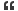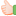1. ##Find the Value

If A+B+C=6 and Q-M = 4
What is the avlue of HXD equal toReply With Quote

2. ## Re: Find the Value

7 x 4 = 28.

-- James.Reply With Quote

3. ## Re: Find the Value

James could you tell me how you got the solution as 28.Reply With Quote

4. ## Re: Find the Value

A = 1
B = 2
C = 3
D = 4
E = 5
F = 6
G = 7
H = 8
-
-
go on..
HXD
hence 8X4 = 32Reply With Quote

5. ## Re: Find the Value

hi,

i agree with jaisri, and i think thats the correct answer.

Thanks
SushmaReply With Quote

6. ## Re: Find the Value

Yes thats the correct answer. Easy oneReply With Quote

7. ## Re: Find the Value

Is it hxd in that x stands for multiply or letter "x"Reply With Quote

8. ## Re: Find the Value

Yes da ans is 8x4=32Reply With Quote

9. ## Re: Find the ValueOriginally Posted by jaisri_10A = 1
B = 2
C = 3
D = 4
E = 5
F = 6
G = 7
H = 8
-
-
go on..
HXD
hence 8X4 = 32
i agree with jaisriReply With Quote

10. ##Re: Find the Value

[QUOTE=timmy;1853]If A+B+C=6 and Q-M = 4
What is the avlue of HXD equal to 768Reply With Quote

11.

####Posting Permissions

• You may not post new threads
• You may not post replies
• You may not post attachments
• You may not edit your posts
•